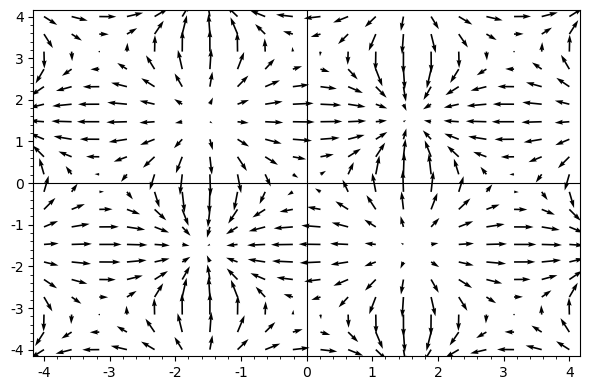# Maxima and Minima¶

The first two images are visualisations of $\sin(x)\cdot sin(y)$ and its gradient.

In :
x, y = var('x y')
P = plot3d(sin(x)*sin(y),(x,-4,4),(y,-4,4))
P

Out:
In :
d = plot_vector_field((sin(y)*cos(x), sin(x)*cos(y)), (x,-4,4), (y,-4,4))
d

Out:# Differentiability¶

The next two graphics illustrate differentiability respectively non-differentiability of the functions $tan(|x|)$ and $|x|$ for $x\in \mathbb R^2$

In :
x, y = var('x y')
o = plot3d(tan(sqrt(x*x+y*y)),(x,-1/2,1/2),(y,-1,1))
o

Out:
In :
x, y = var('x y')
a = plot3d((sqrt(x*x+y*y)),(x,-1/2,1/2),(y,-1,1))
a

Out:

# No Converse to Schwarz theorem¶

The function $\frac{x^2y^2}{x^2+y^2}$ has commuting mixed partial derivatives but the mixed partial derivative (plotted below) is not continuous.

In :
x, y = var('x y')
P = plot3d(8*x^3*y^3/(x^2+y^2)^3 ,(x,-4,4),(y,-4,4))
P

Out:

# A travelling wave¶

A sample "sketch" of a solution of the 1-D wave equation. (The capped parabola should be preferrably substitued with something at least twice differentiable).

In :
phii(y)=max(1-y^2,0)*heaviside(1-y)*heaviside(1+y)
wave = [plot((phii(x-t)), (-4,4),  ymin=-1, ymax=1) for t in sxrange(-3,3,.2)]
a = animate(wave)
a

Out: